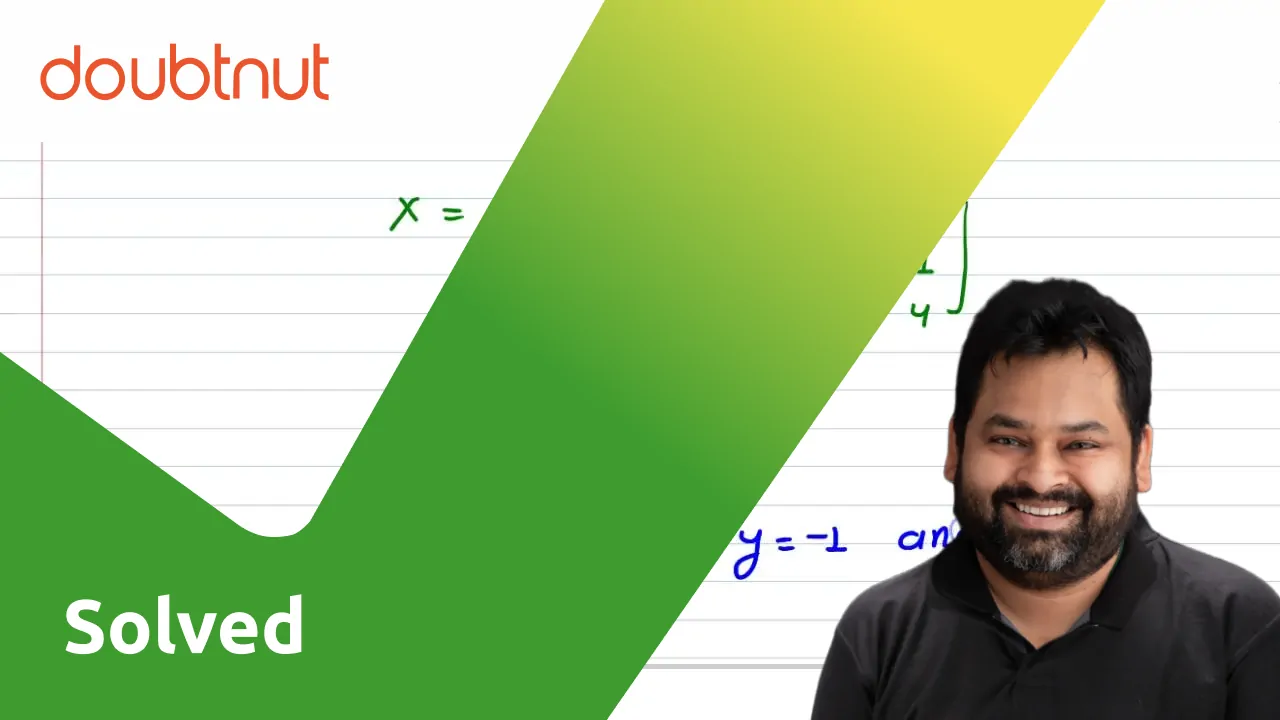Doubtnut App - Get 50 Lakh+ video solutions better than GoogleInstall Now

# Evaluate the product AB , where A=⎡⎢⎣1−10234012⎤⎥⎦andB=⎡⎢⎣22−4−42−42−15⎤⎥⎦ hence solve the system of linear equations x−y=3 2x+3y+4z=17 y+2z=7

Video Solution